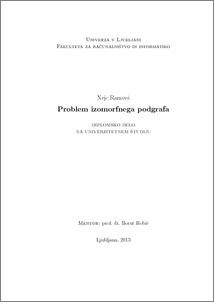# The subgraph isomorphism problem

Nejc Ramovš (2013) The subgraph isomorphism problem. EngD thesis.Preview
PDF

## Abstract

This thesis describes the problem of finding subgraph isomorphism. This is one of the most basic operations performed on graphs and is an NP-hard problem. We describe in detail the Ullmann algorithm and VF2 algorithm, the most commonly used and state-of-the art algorithms in this field, and a new algorithm called Subsea. We improved the VF2 algorithm using some principles from Subsea. The improvement was implemented in C++ and then compared against VF2 algorithm implementation from the program library vflib and an implementation of improved Ullmann algorithm. We tested the algorithms on a database of 9000 pairs of randomly generated graphs. In compariton to the VF2 algorithm the results show a speedup factor of at least 5 for the improved version of Ullmann algorithm and a speedup factor of at least 30 for the improved version of VF2 algorithm. The improved version of Ullmann algorithm was the fastest algorithm for small graphs, while for large graphs and graphs with many connections out improved version of VF2 algorithm proved the fastest.

Item Type: Thesis (EngD thesis)
Keywords: graph, subgraph, isomorphism, algorithm
Number of Pages: 52
Language of Content: Slovenian
Mentor / Comentors:
Name and SurnameIDFunction
prof. dr. Borut Robič28Mentor